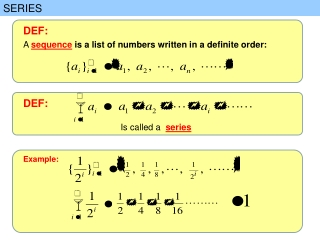DownloadDownload PresentationSERIES

# SERIES

Download Presentation## SERIES

- - - - - - - - - - - - - - - - - - - - - - - - - - - E N D - - - - - - - - - - - - - - - - - - - - - - - - - - -
##### Presentation Transcript

1. SERIES DEF: A sequence is a list of numbers written in a definite order: DEF: Is called a series Example:

2. SERIES DEF: Is called a series Example: its sum n-th term DEF: If the sum of the series convergent is finite number not infinity

3. SERIES Example: DEF: Given a seris nth-partial sums : DEF: Given a seris the sequence of partial sums. :

4. SERIES We define Given a series Sequence of partial sums Given a series Sequence of partial sums

5. SERIES We define Given a series Sequence of partial sums DEF: If convergent convergent If divergent divergent

6. SERIES Example:

7. SERIES Special Series: Geometric Series: • Geometric Series • Harmonic Series • Telescoping Series • p-series • Alternating p-series first term Common ratio Example Example: Example Is it geometric? Is it geometric?

8. SERIES Geometric Series: Geometric Series: Example: Example Example Is it geometric?

9. SERIES Final-111

10. SERIES Final-102

11. SERIES Geometric Series: Geometric Series: prove:

12. SERIES Geometric Series: Geometric Series:

13. SERIES Final-102

14. SERIES Geometric Series: Geometric Series:

15. SERIES Special Series: Telescoping Series: • Geometric Series • Harmonic Series • Telescoping Series • p-series • Alternating p-series Telescoping Series: Convergent Convergent Example: Remark: b1 means the first term ( n starts from what integer)

16. SERIES Telescoping Series: Convergent Convergent Final-111

17. SERIES Telescoping Series: Telescoping Series: Convergent Convergent Notice that the terms cancel in pairs. This is an example of a telescoping sum: Because of all the cancellations, the sum collapses (like a pirate’s collapsing telescope) into just two terms.

18. SERIES Final-101 Final-112

19. SERIES Final-101

20. SERIES THEOREM: Convergent THEOREM:THE TEST FOR DIVERGENCE Divergent

21. SERIES THEOREM:THE TEST FOR DIVERGENCE Divergent

22. SERIES THEOREM:THE TEST FOR DIVERGENCE Divergent THEOREM: Convergent REMARK(1): The converse of Theorem is not true in general. If we cannot conclude that is convergent. Convergent REMARK(2): the set of all series If we find that we know nothing about the convergence or divergence

23. SERIES THEOREM: Convergent REMARK(2): Seq. series REMARK(3): Sequence convg convg

24. SERIES REMARK Example All these items are true if these two series are convergent

25. SERIES Final-082

26. SERIES Final-081

27. SERIES Final-092

28. SERIES Final-121

29. SERIES Final-121

30. SERIES Final-103

31. SERIES

32. SERIES Adding or Deleting Terms Example REMARK(4): A finite number of terms doesn’t affect the divergence of a series. REMARK(5): Example A finite number of terms doesn’t affect the convergence of a series. REMARK(6): A finite number of terms doesn’t affect the convergence of a series but it affect the sum.

33. SERIES Reindexing Example We can write this geometric series

34. SERIES Special Series: • Geometric Series • Harmonic Series • Telescoping Series • p-series • Alternating p-series

35. summary SERIES THEOREM:THE TEST FOR DIVERGENCE Divergent convg convg

36. SERIES Geometric Series: Geometric Series: Example Write as a ratio of integers

37. SERIES Final-101

38. SERIES Final-112